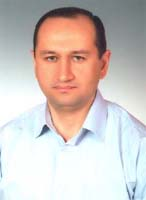## Dr. Azer Hanmehmetli (Khanmamedov)

(Professor of Applied Mathematics)

Phone: +90 0 312 2977865, Office: 246

E-Mail: azer@hacettepe.edu.tr

Research Interests:
Nonlinear partial differential equations; Attractors of evolution equations.
Publications

### Research Papers:

1. Attractors for some models of the Cahn-Hilliard equation with the inertial term // Nonlinearity, 36 (2023), 1120-1142.
2. On the two dimensional fast phase transition equation: well-posedness and long-time dynamics // Calculus of Variations and Partial Differential Equations, 60 (2021), 200.
3. On the 2D Cahn-Hilliard/Allen-Cahn equation with the inertial term // J. Math. Anal. Appl., 494 (2021), 124603.
4. Long-time dynamics of the Swift-Hohenberg equations // J. Math. Anal. Appl., 483 (2020), 123626.
5. Global attractors for the 2D hyperbolic Cahn-Hilliard equations // Z. Angew. Math. Phys., 69(1)(2018), 1-17 (with S. Yayla).
6. Long-time dynamics of the strongly damped semilinear plate equation in Rn // Acta Math. Sci., 38(2018), 1025-1042 (with S. Yayla).
7. Attractors for the strongly damped wave equation with p-Laplacian // Mathematical Methods in the Applied Sciences, 40 (2017), 4436-4447 (with Z. Sen).
8. Existence of the global attractor for the plate equation with nonlocal nonlinearity in Rn // Discrete and Continuous Dynamical Systems-B, 21 (2016), 151-172 (with S. Simsek).
9. A unique continuation result for the palate equation and an application // Mathematical Methods in the Applied Sciences, 39 (2016), 744-761 (with Z. Arat and S. Simsek).
10. Exponential decay of solutions for the plate equation with localized damping // Mathematical Methods in the Applied Sciences, 38 (2015), 1767-1780 (with S. Simsek).
11. Global attractors for the plate equation with nonlocal nonlinearity in unbounded domains // Dyn. Partial Differ. Equations, 11 (2014), 361379 (with Z. Arat and S. Simsek).
12. Strongly damped wave equation with exponential nonlinearities // J. Math. Anal. Appl., 419 (2014), 663687.
13. Long-time dynamics of the parabolic p-Laplacian equation // Communications on Pure and Applied Analysis, 12 (2013), 735-754 (with P.G. Geredeli).
14. Global attractor for the one dimensional wave equation with displacement dependent damping // Applied Mathematics Letters, 25 (2012), 439-442.
15. Global attractors for strongly damped wave equations with displacement dependent damping and nonlinear source term of critical exponent // Discrete and Continuous Dynamical Systems, 31 (2011), 119-138.
16. A global attractor for the plate equation with displacement-dependent damping // Nonlinear Analysis: TMA, 74 (2011), 1607-1615.
17. On the long-time behavior of the quasilinear parabolic equation // AIP Conf. Proc. 1281 (2010), 1987-1990 (with P.G. Geredeli).
18. A strong global attractor for the 3D wave equation with displacement dependent damping // Applied Mathematics Letters, 23 (2010), 928-934.
19. Remark on the regularity of the global attractor for the wave equation with nonlinear damping // Nonlinear Analysis: TMA, 72 (2010), 1993-1999.
20. Global attractors for 2-D wave equations with displacement-dependent damping // Mathematical Methods in the Applied Sciences, 33 (2010), 177-187.
21. On the a global attractor for the strong solutions of the 2-D wave equation with nonlinear damping // Applicable Analysis, 88 (2009), 1283-1301.
22. Global attractors for one dimensional p-Laplacian equation // Nonlinear Analysis: TMA, 71 (2009), 155-171.
23. Long-time behaviour of doubly nonlinear parabolic equations // Communications on Pure and Applied Analysis, 8 (2009), 1373-1400.
24. On the existence of a global attractor for the wave equation with nonlinear strong damping perturbed by nonmonotone term // Nonlinear Analysis: TMA, 69 (2008), 3372-3385.
25. Long-time behaviour of wave equations with nonlinear interior damping // Discrete and Continuous Dynamical Systems, 21 (2008), 1185-1998.
26. Finite dimensionality of the global attractors for von Karman equations with nonlinear interior dissipations // Nonlinear Analysis: TMA, 66 (2007), 204-213.
27. Global attractors for wave equations with nonlinear interior damping and critical exponents // J. Differential Equations, 230 (2006), 702-719.
28. Global attractors for the plate equation with a localized damping and a critical exponent in an unbounded domain // J. Differential Equations, 225 (2006), 528-548.
29. Global attractors for von Karman equations with nonlinear interior dissipation // J. Math. Anal. Appl., 318 (2006), 92-101.
30. Existence of a global attractor for the parabolic equation with non-linear Laplacian principal part in an unbounded domain // J. Math. Anal. Appl., 316 (2006), 601-615.
31. Existence of a global attractor for the plate equation with critical exponent in unbounded domain // Applied Mathematics Letters, 18 (2005), 827-832.
32. Existence of a global attractor for the semi-linear plate equation in unbounded domain // Transaction of NAS Azerbaijan, XXII (2002), 144-150.
33. Stabilization of solution for the semi-linear wave equation // Transactions of NAS Azerbaijan, XX (2000), 55-59 .
34. The existence of the attractor for the semi-linear wave equation with localized damping // Proceedings of Institute Mathematics and Mechanics, XIX (1999), 89-95.
35. On the existence of minimal global attractor for the nonlinear wave equation with antidissipation inside the domain and dissipation on a part of the boundary // Differential Equations, 34 (1998), 326-330 (with A.B. Aliev).
36. Energetic estimates of solutions of the mixed problem for the second order linear hyperbolic equations // Mathematical Notes, 59 (1996), 483-488 (with A.B. Aliev).
Conferences and Meetings
• IECMSA, Vienna, Austria, 2014.
• IECMSA, Saraejevo, Bosnia-Herzegovina, 2013.
• Workshop on Dynamical Systems, Gebze - Tüsside, 2011.
• Workshop on Dynamical Systems, Izmir University, 2009.
• Workshop on Differential Equations, Yeditepe University, 2008.
• ANKARA MATHEMATICAL DAYS, PDE MEETINGS VI, Çankaya University , 2006.
• ANKARA MATHEMATICAL DAYS, PDE MEETINGS V, Hacettepe University, 2005.
• VIII Republic Conference on Mathematics and Mechanics, Baku , 2002.
• I Republic Conference on Mathematics and Mechanics, Baku , 1995.
• XI Republic Conference of Young Scientists on Mathematics and Mechanics, Baku , 1994.
Courses Given

Real Analysis, Partial Differential Equations, Functional Analysis, Analysis III, Analysis IV, Ordinary Differantial Equations.